# OpenCV 中的轮廓

## 轮廓：入门

### 什么是轮廓？

import numpy as np
import cv2 as cv
imgray = cv.cvtColor(im, cv.COLOR_BGR2GRAY)
ret, thresh = cv.threshold(imgray, 127, 255, 0)
contours, hierarchy = cv.findContours(thresh, cv.RETR_TREE, cv.CHAIN_APPROX_SIMPLE)


### 如何绘制轮廓？

• 要在图像中绘制所有轮廓：
cv.drawContours(img, contours, -1, (0,255,0), 3)

• 要绘制单个轮廓，请说第四个轮廓：
cv.drawContours(img, contours, 3, (0,255,0), 3)

• 但是在大多数情况下，以下方法会很有用：
cnt = contours
cv.drawContours(img, [cnt], 0, (0,255,0), 3)


### 轮廓近似法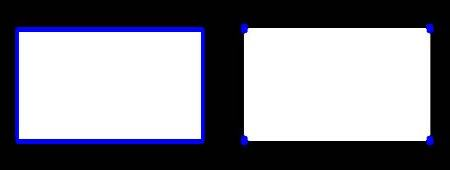## 轮廓特征

### 1. 矩

import numpy as np
import cv2 as cv
ret,thresh = cv.threshold(img,127,255,0)
contours,hierarchy = cv.findContours(thresh, 1, 2)
cnt = contours
M = cv.moments(cnt)
print( M )


cx = int(M['m10']/M['m00'])
cy = int(M['m01']/M['m00'])


### 2.轮廓面积

area = cv.contourArea(cnt)


### 3.轮廓周长

perimeter = cv.arcLength(cnt,True)


### 4.轮廓近似

epsilon = 0.1*cv.arcLength(cnt,True)
approx = cv.approxPolyDP(cnt,epsilon,True)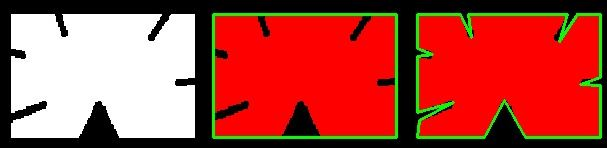### 5.凸包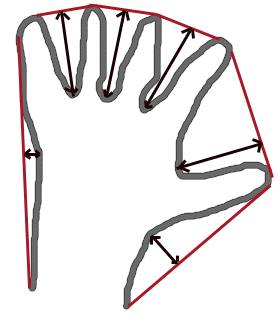hull = cv.convexHull(points[, hull[, clockwise[, returnPoints]]])


• points: 就是我们传入的轮廓。
• hull: 是输出，通常我们避免它。
• clockwise：方向标记。如果为True，则输出凸包为顺时针方向。否则，其方向为逆时针方向。
• returnPoints：默认情况下为True。然后返回船体点的坐标。如果为False，则返回与船体点相对应的轮廓点的索引。

hull = cv.convexHull(cnt)


### 6.检查凸度

cv.isContourConvex() 是一个函数用来检查曲线是否为凸多边形。它只是返回True还是False。

k = cv.isContourConvex(cnt)


### 7.边界矩形

#### 7.a. 直角矩形

x,y,w,h = cv.boundingRect(cnt)
cv.rectangle(img,(x,y),(x+w,y+h),(0,255,0),2)


#### 7.b．旋转矩形

rect = cv.minAreaRect(cnt)
box = cv.boxPoints(rect)
box = np.int0(box)
cv.drawContours(img,[box],0,(0,0,255),2)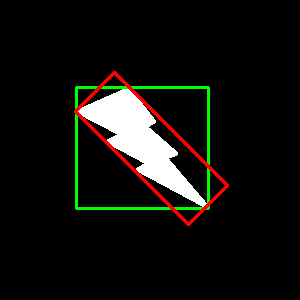### 8.最小外圆

(x,y),radius = cv.minEnclosingCircle(cnt)
center = (int(x),int(y))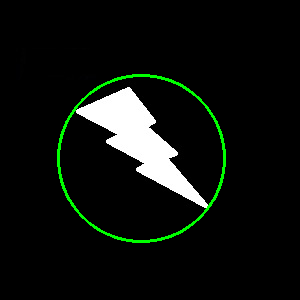### 9.拟合椭圆

ellipse = cv.fitEllipse(cnt)
cv.ellipse(img,ellipse,(0,255,0),2)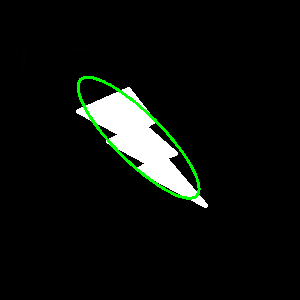### 10.拟合线

rows,cols = img.shape[:2]
[vx,vy,x,y] = cv.fitLine(cnt, cv.DIST_L2,0,0.01,0.01)
lefty = int((-x*vy/vx) + y)
righty = int(((cols-x)*vy/vx)+y)
cv.line(img,(cols-1,righty),(0,lefty),(0,255,0),2)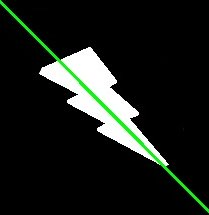## 轮廓属性

(注意：质心，面积，周长等也属于此类，但我们在上一章中已经看到了)

### 1.长宽比

x,y,w,h = cv.boundingRect(cnt)
aspect_ratio = float(w)/h


### 2.范围

area = cv.contourArea(cnt)
x,y,w,h = cv.boundingRect(cnt)
rect_area = w*h
extent = float(area)/rect_area


### 3.固实性

$Solidity = \frac{Contour \; Area}{Convex \; Hull \; Area}$
area = cv.contourArea(cnt)
hull = cv.convexHull(cnt)
hull_area = cv.contourArea(hull)
solidity = float(area)/hull_area


### 4.等效直径

area = cv.contourArea(cnt)
equi_diameter = np.sqrt(4*area/np.pi)


### 5.方向

(x,y),(MA,ma),angle = cv.fitEllipse(cnt)


### 6.遮罩和像素点

mask = np.zeros(imgray.shape,np.uint8)


### 7.最大值，最小值及其位置

min_val, max_val, min_loc, max_loc = cv.minMaxLoc(imgray,mask = mask)


### 8.平均颜色或平均强度

mean_val = cv.mean(im,mask = mask)


### 9.极端点

leftmost = tuple(cnt[cnt[:,:,0].argmin()])
rightmost = tuple(cnt[cnt[:,:,0].argmax()])
topmost = tuple(cnt[cnt[:,:,1].argmin()])
bottommost = tuple(cnt[cnt[:,:,1].argmax()])## 轮廓：更多功能

### 目标

• 凸性缺陷以及如何找到它们。
• 查找点到多边形的最短距离
• 匹配不同的形状

### 理论和代码

#### 1.凸包缺陷

OpenCV带有一个现成的函数 cv.convexityDefect() 来查找该函数。基本的函数调用如下所示：

hull = cv.convexHull(cnt,returnPoints = False)
defects = cv.convexityDefects(cnt,hull)


import cv2 as cv
import numpy as np
img_gray = cv.cvtColor(img,cv.COLOR_BGR2GRAY)
ret,thresh = cv.threshold(img_gray, 127, 255,0)
contours,hierarchy = cv.findContours(thresh,2,1)
cnt = contours
hull = cv.convexHull(cnt,returnPoints = False)
defects = cv.convexityDefects(cnt,hull)
for i in range(defects.shape):
s,e,f,d = defects[i,0]
start = tuple(cnt[s])
end = tuple(cnt[e])
far = tuple(cnt[f])
cv.line(img,start,end,[0,255,0],2)
cv.circle(img,far,5,[0,0,255],-1)
cv.imshow('img',img)
cv.waitKey(0)
cv.destroyAllWindows()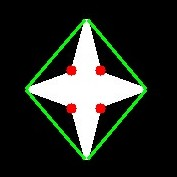#### 2.点多边形测试

dist = cv.pointPolygonTest(cnt,(50,50),True)


#### 3.匹配形状

OpenCV带有函数 cv.matchShapes()，使我们能够比较两个形状或两个轮廓，并返回显示相似性的度量。结果越低，匹配越好。它是基于 hu-moment 值计算的。文档中介绍了不同的测量方法。

import cv2 as cv
import numpy as np
ret, thresh = cv.threshold(img1, 127, 255,0)
ret, thresh2 = cv.threshold(img2, 127, 255,0)
contours,hierarchy = cv.findContours(thresh,2,1)
cnt1 = contours
contours,hierarchy = cv.findContours(thresh2,2,1)
cnt2 = contours
ret = cv.matchShapes(cnt1,cnt2,1,0.0)
print( ret )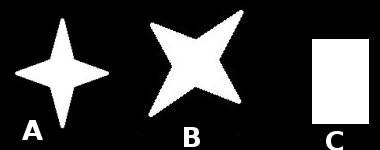### 练习题

1. 检查文档 cv.pointPolygonTest() ，您可以找到红色和蓝色的漂亮图像。它表示从所有像素到其上的白色曲线的距离。曲线内的所有像素均为蓝色，具体取决于距离。同样，外部点为红色。等高线边缘用白色标记。所以问题很简单。编写代码以创建距离的这种表示。
2. 使用 cv.matchShapes() 比较数字或字母的图像。(那将是迈向OCR的简单步骤)

## 轮廓层次

### 理论

#### 什么是层次结构？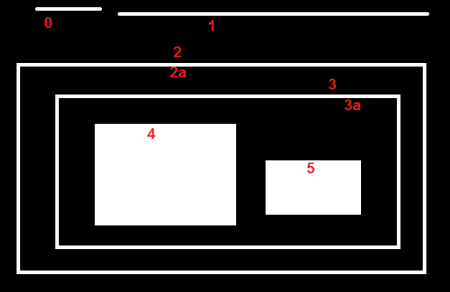#### OpenCV中的层次结构表示

Next 表示相同等级的下一个轮廓 例如，在我们的图片中选择轮廓0。谁是同一级别的下一个轮廓？它是轮廓1。因此，只需将Next = 1放进去。同样对于Contour-1，下一个就是轮廓线2。所以下一个= 2。

Previous 表示相同轮廓级别的上一个轮廓 和上面一样。轮廓1的先前轮廓是同一级别的轮廓0。同样对于轮廓2，它是轮廓1。对于轮廓0，没有先前值，因此将其设为-1。

First_Child 表示其第一个子轮廓 无需任何解释。对于轮廓2，子级是轮廓2a。这样就得到了轮廓2a的相应索引值。那轮廓3a呢？它有两个孩子。但是我们只带第一个孩子。它是轮廓4。因此，轮廓3a的First_Child = 4。

Parent 代表示其父代轮廓的索引 它与First_Child相反。轮廓4和轮廓5的父轮廓均为轮廓3a。对于轮廓3a，它是轮廓3，依此类推。

#### 轮廓检索模式

##### 1. RETR_LIST

>>> hierarchy
array([[[ 1, -1, -1, -1],
[ 2,  0, -1, -1],
[ 3,  1, -1, -1],
[ 4,  2, -1, -1],
[ 5,  3, -1, -1],
[ 6,  4, -1, -1],
[ 7,  5, -1, -1],
[-1,  6, -1, -1]]])


##### 2. RETR_EXTERNAL

>>> hierarchy
array([[[ 1, -1, -1, -1],
[ 2,  0, -1, -1],
[ 3,  1, -1, -1],
[ 4,  2, -1, -1],
[ 5,  3, -1, -1],
[ 6,  4, -1, -1],
[ 7,  5, -1, -1],
[-1,  6, -1, -1]]])


##### 3. RETR_CCOMP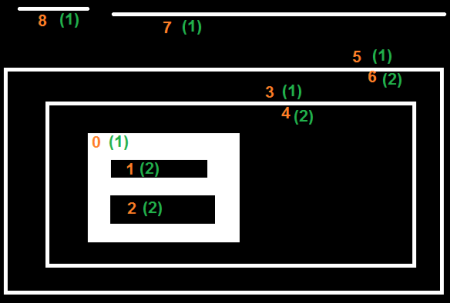>>> hierarchy
array([[[ 3, -1,  1, -1],
[ 2, -1, -1,  0],
[-1,  1, -1,  0],
[ 5,  0,  4, -1],
[-1, -1, -1,  3],
[ 7,  3,  6, -1],
[-1, -1, -1,  5],
[ 8,  5, -1, -1],
[-1,  7, -1, -1]]])

##### 4. RETR_TREE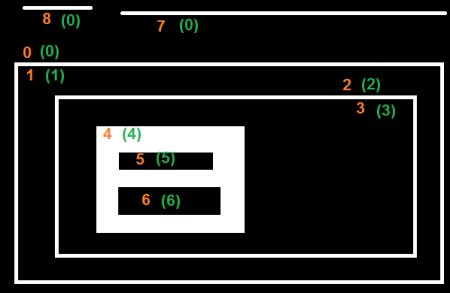>>> hierarchy
array([[[ 7, -1,  1, -1],
[-1, -1,  2,  0],
[-1, -1,  3,  1],
[-1, -1,  4,  2],
[-1, -1,  5,  3],
[ 6, -1, -1,  4],
[-1,  5, -1,  4],
[ 8,  0, -1, -1],
[-1,  7, -1, -1]]])